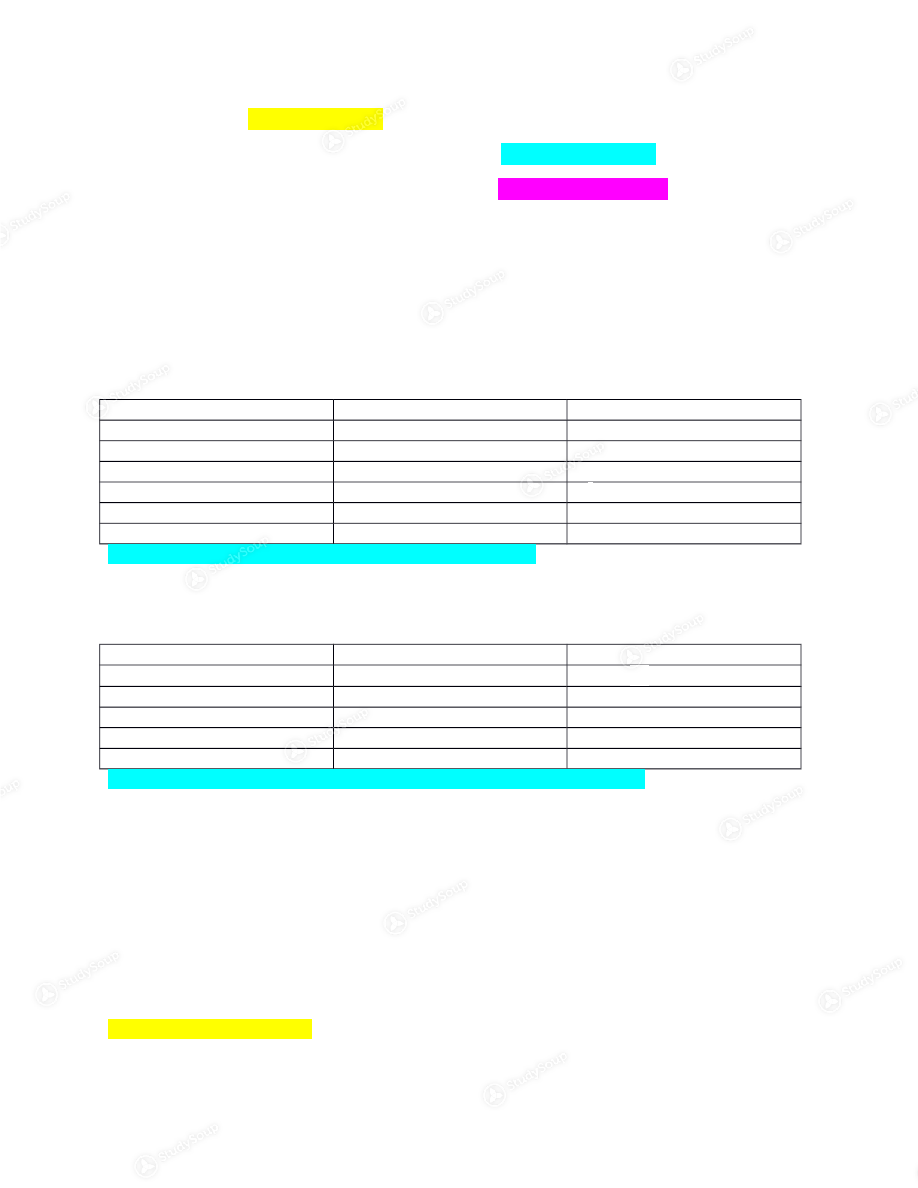Limited time offer 20% OFF StudySoup Subscription details

# CSU - CHEM 111 - Chem 111 Week 2 notes - Class Notes

### Created by: Kristine Emken Elite Notetaker

> > > > CSU - CHEM 111 - Chem 111 Week 2 notes - Class Notes

CSU - CHEM 111 - Chem 111 Week 2 notes - Class Notes

0 5 3 57 Reviews
This preview shows pages 1 - 3 of a 11 page document. to view the rest of the contentDefinitions will be highlighted yellow Reminders and extra important things to know will be highlighted light blue with *stars* Other basic information that you should know will be highlighted light purple 1.4 Measurements International system of units (SI) is the metric system which has decimally related units These are the major and commonly used units Length Meter m Mass Kilograms kg Temperature Kelvin K Time Seconds s Volume Cubic meters or Liters m ³  or L Quantity of a substance Mole mol Electric current Ampere A *Note you won’t be using A for the electric current in this class* Metric prefixes  Mega M 1Mm=10 m Kilo k 1km=10 ³m Centi c 1cm=10 ²m Milli m 1mm=10 ³m Micro µ 1µm=10 m ⁻⁶ Nano n 1nm=10 m ⁻⁹ **You will need to know all of these and memorize them before the first exam** Density­ ratio of mass to volume (D=M/V) 1.5 Measurement Uncertainty, Accuracy, and Precision Exact numbers­ defined values Ex: Things you can count (12 eggs)Ex: Defined values (1kg=1000g) *If a number is exact you do not consider it for significant figures* Inexact numbers­ have some degree of uncertainty Ex: Measured quantities (the table is 17.5” wide) To express the uncertainty, we use significant figures. The significant figures will show how  accurately we know the number. *The last digit in a number is where the uncertainty lies (17.5” the 5 after the decimal is the  uncertain digit)* Rules for significant figures in a reported measurement 1. Nonzero digits are significant (1,2,3,4… 9) 2. Zeros between two significant digits are significant (207, the 0 between the 2 and the 7 is  significant) 3. Zeros to the lefts are NOT significant (06 the 6 is the only significant digit) (0.002, the 2 is the  only significant digit) 4. Zeros at the end (to the right) are significant ONLY if there is a decimal point (0.060, the 6 and  the 0 to the right are significant due to the decimal) (30., all of these are significant due to the
decimal) (4.0, both are significant because the 0 is to the right and there is a decimal)
Significant figure rules Multiplication/Division Answers are rounded to the least number of significant figures Ex: V= 14.5”x22.34”x7.0” =2267.51in³ 14.5” has 3 significant figures, 22.34” has 4 significant figures, and 7.0”  has 2 significant figures (*remember 0s to the right are important if there is a decimal  place*). Since we are multiplying we will use the least number of significant  figure which is 2. The answer would be 2200in³ *remember the 0s to the right aren’t important  unless there is a decimal point. Or you could write the answer in scientific notation which would
be 2.2x10³
Addition/Subtraction Answers are rounded to the least significant figure to the left most column after the  decimal place (or to the least significant digit to the right of the decimal place) Ex: 9.101+3.25+7.9=20.251A better way to write this would be     9.1 | 01     3.2 | 5   +7.9 | =20.2 | 51 Now we look at the rule where you look at the digit in the left most column after  the decimal place, shown by the line between the digits in the tenth and hundredths place. This is
the column with a digit furthest to the left, after the decimal. This is where we would round.
The answer would be: 20.3 (due to the .05 having to round up) Multiple steps Through the different steps keep track of the number of significant figures per step and  round ONLY at the end. *It is helpful if you do each step individually* Ex:   1.656 g−1.54 g 0.1000 mL First, we will do the subtraction in the numerator which gives us  0.116 g 0.1000 mL **Keep track of how many significant digits WOULD be in the numerator for  the addition/subtraction rule if we did not have another step** The 0.116g would be 0.12g After the division the answer would be 1.16 g/mL Remember what was said  above about what the numerator would be with the addition/subtraction rule which is the 0.12g so
the least number of significant figures would be 2 (from 0.12g). This would make the final
Precision­ reproducible results/ results relatively close together (hitting the same place on a target)

This is the end of the preview. Please to view the rest of the contentJoin more than 18,000+ college students at Colorado State University who use StudySoup to get ahead
##### Description: -Metric system units -Metric prefixes -Exact vs. inexact numbers -Uncertainty in numbers -Precision vs. accuracy -Rules for significant figures in measurements -Rules for significant figures with multi
11 Pages 45 Views 36 Unlocks
• Notes, Study Guides, Flashcards + More!
Get Full Access to CSU - Chem 111 - Class Notes - Week 2
×
Get Full Access to CSU - Chem 111 - Class Notes - Week 2

I don't want to reset my password

Need help? Contact support

Need an Account? Is not associated with an account
We're here to help